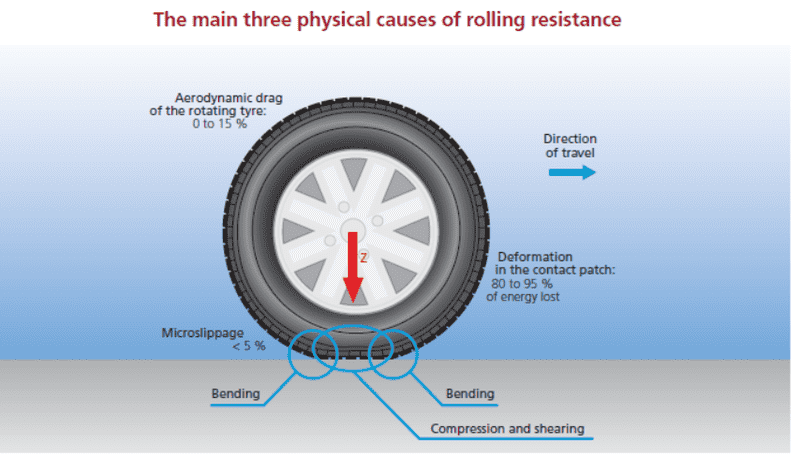# Can we calculate the rolling resistance coefficient theoretically?

• Souhardya Nandi

#### Souhardya Nandi

I was wondering that if rolling resistance is basically caused by slight deformation of a body, we must have formulas in place to relate it to the physical and material properties of a body. But when I went ravaging for it in library or internet, everything was experimental. I wished to know from all fellow PFians if you have knowledge of any formula which can calculate the rolling resistance coefficient of a body from elasticity,radius,density, etc. without any experimentation whatsoever ?

Last edited:

What's rolling friction? There is static friction (not slipping), dynamic friction (slipping), and rolling resistance (deformation of the rolling body as it moves)...

What's rolling friction? There is static friction (not slipping), dynamic friction (slipping), and rolling resistance (deformation of the rolling body as it moves)...
You misunderstood my question, sir. I am not asking what rolling friction is. I am asking if there is an existing formula to find the rolling friction coefficient of a body from its material properties without any form of experimentation. Do reply if you can.

You misunderstood my question, sir. I am not asking what rolling friction is. I am asking if there is an existing formula to find the rolling friction coefficient of a body from its material properties without any form of experimentation. Do reply if you can.
I understood the question, but your terminology may have a problem. There is no such thing as rolling friction (except for bearing friction maybe). Do you mean that you want to understand if Rolling Resistance can be calculated?